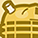# New to Qlik Sense

If you’re new to Qlik Sense, start with this Discussion Board and get up-to-speed quickly.

Announcements
An issue has been identified on Qlik Cloud hub, please visit our Status Update Page for details: GET THE LATEST
cancel
Showing results for
Did you mean:Contributor III

## Number of Zeros after a non Zero number

Guys, I have a column with transaction values.

The business case is to count the number of zeros starting from the right side of the transaction value before a non zero number. For example,

if the transaction value is 10 then number of zero should be 1, if the transaction value is 10,000 then number of zero should be 4, if the transaction value is 10, 500 then number of zero should be 2 (as the last two digits contains 2 zeros).

 Transaction Value Number of Zeros 10 1 100 2 1,000 3 10,500 2 20,253 0

Is this possible to do in Qliksense?

1 Solution

Accepted SolutionsMVP

Sorry, that should be mod = 0, not >0 (I got caught halfway between using mod and using frac, hence the mistake).

Another note - you didn't specify what should happen if the value is exactly 0, so you may want to add an if() statement to handle that specific case if 0 is a possible value.

4 RepliesMVP

You could simply nest some if() statements and use Mod(Value,10), Mod(Value,100) etc. if your numbers don't get too big, e.g.

If(Mod(Value,10000)=0,4,if(Mod(Value,1000)=0,3,if(Mod(Value,100)=0,2,if(Mod(Value,10)=0,1,0))))

It's not particularly clean, but it should work, and odds are your numbers aren't going to get so big that this won't work.Contributor III
Author

I tried the code but I'm not getting the desired results. For Transaction Value 12,339 the number of zero column populates the count as 4,  Transaction Value 7,020 the number of zero column populates the count as 4.MVP

Sorry, that should be mod = 0, not >0 (I got caught halfway between using mod and using frac, hence the mistake).

Another note - you didn't specify what should happen if the value is exactly 0, so you may want to add an if() statement to handle that specific case if 0 is a possible value.Contributor III
Author

0 is not a possible value so works perfectly. Thank you so muchTags
Community Browser